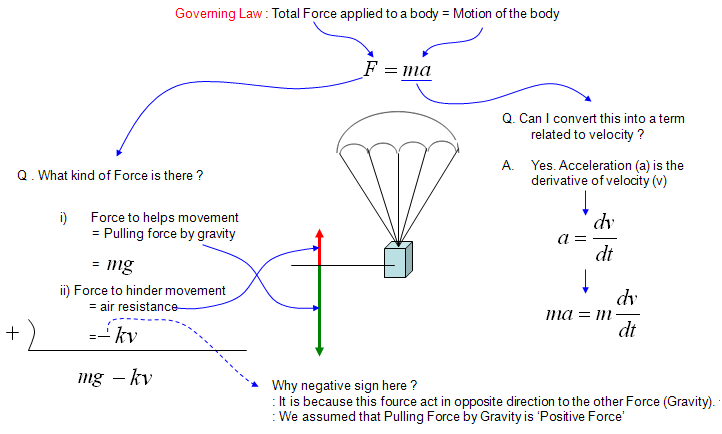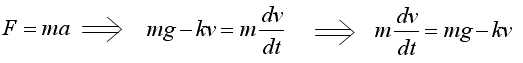DE - Modeling                                                                          Home : www.sharetechnote.com Velocity of Freefall Body   Direction : Derive a differential equation that tells you the velocity of a falling body at any given time. (Assume the condition where you should not ignore the air resistance)   What is the governing rule ? Total Force applied to a body = Motion of the bodyNote : This can be one of the simplest modeling example, but it doesn't mean that you should be able to understand everything here in 5 minutes and you are dumb if you don't understand this so quickly. I hope you would have a lot of questions that has not clearly been explained in my explanation above and that has not been treated in your math course. For example, my own questions are like this. The explanation said the air resistance can be expressed as -kv, but how did you know that ? Jugding from the expression, it seems that the air resistance is determined only by the velocity ? How did you guarantee that the resistance is not influenced by other factors like acceleration, mass of the falling body ?  How about the shape of the falling body or paraschute ? Doesn't this influence the resistance force ? Judging from our common sense, the shape/size of the paraschute definately influence the resistance force. How come this term is not shown in the mathematical expression, -kv ?  what does 'k' mean ? How can we determine this value ? what kind of factors are involved in determining k value (Actually, the shape of paraschute or falling body can be a very important factors to determine the k value). Like this, we can have an endless list of questions boggling in your mind if you are really doing the mathematical modeling in stead of rotely memorizing whatever your textbook or teach tells. I am not saying that you always have to raise your hand in your math class and argue with your math teacher until you get the answers to all of these kind of questions. These are the questions you continue to investigate to expand this kind of basic model to a better/real life models.